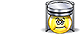Unit Conversion Software Web Widgets Loan Calculator Currency Rates Country Flags Unit Converter
 Faq HelpDecipascal ConversionContact

Decipascal, unit of measure

To perform conversions between decipascal and other Pressure units please try our Pressure Unit Converter

Convert decipascal to:
pascal, exapascal, petapascal, terapascal, gigapascal, megapascal, kilopascal, hectopascal, dekapascal, centipascal, millipascal, micropascal, nanopascal, picopascal, femtopascal, attopascal, newton/square meter, newton/square centimeter, newton/square millimeter, kilonewton/square meter, bar, millibar, microbar, dyne/square centimeter, kilogram-force/square meter, kilogram-force/square centimeter, kilogram-force/square millimeter, gram-force/square centimeter, ton-force (short)/square foot, ton-force (short)/square inch, ton-force (long)/square foot, ton-force (long)/square inch, kip-force/square inch, ksi, pound-force/square foot, pound-force/square inch, psi, poundal/square foot, torr, centimeter mercury (0°C), millimeter mercury (0°C), inch mercury (32°F), inch mercury (60°F), centimeter water (4°C), millimeter water (4°C), inch water (4°C), foot water (4°C), inch water (60°F), foot water (60°F), atmosphere technical, Standard atmosphereDownload Pressure Unit Converter
our powerful software utility that helps you make easy conversion between more than 2,100 various units of measure in more than 70 categories. Discover a universal assistant for all of your unit conversion needs - download the free demo version right away!Copyright © 1998-2009 UnitConversion.org Privacy & Terms | About | Faq | Help | Contact | Link to Us